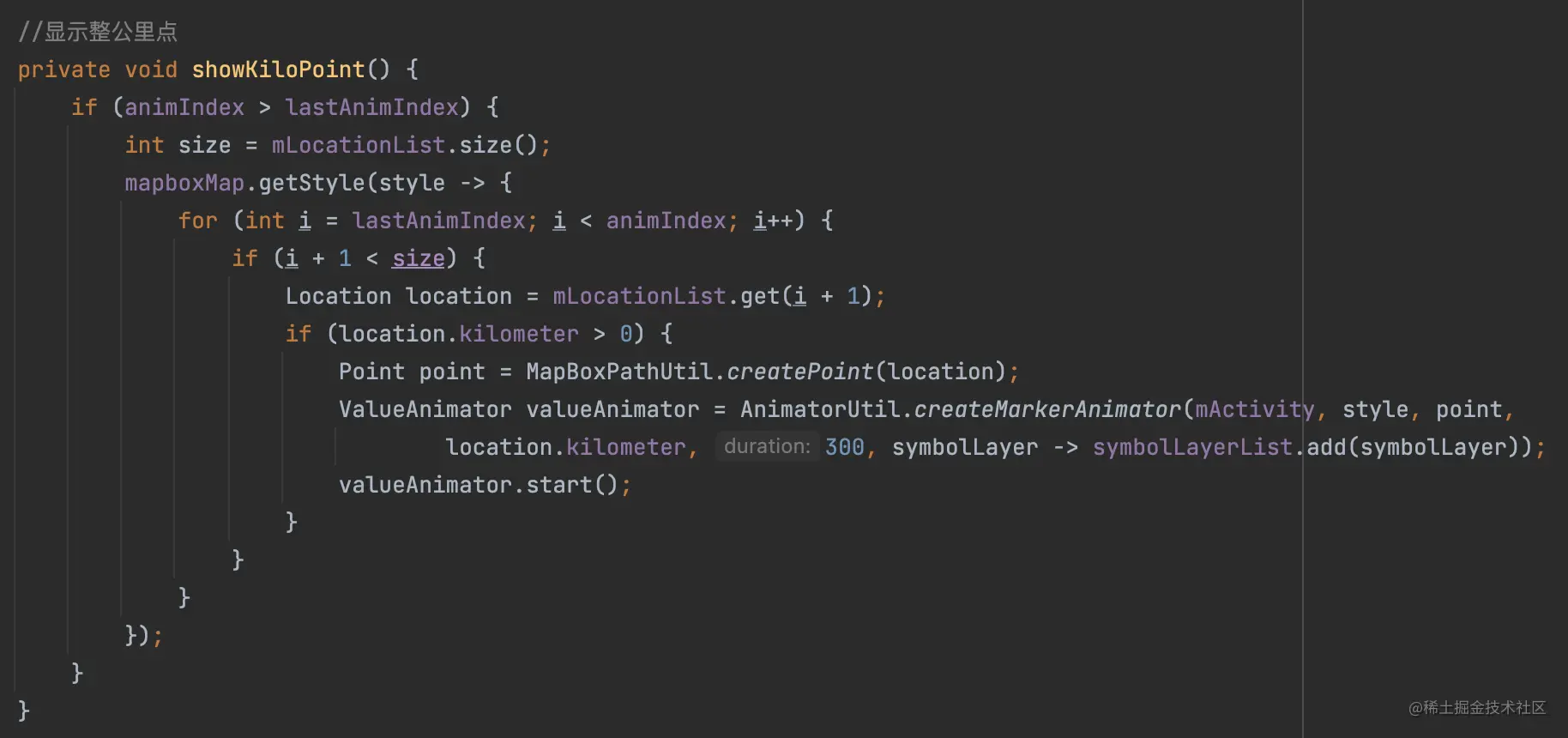# 运动APP视频轨迹回放分享实现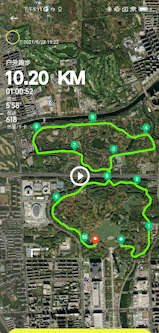#### 基于Path Length的属性动画

1. 计算List对应的Path
2. 通过PathMeasure获取 Path 的 Length
3. 对Path做 Length的属性动画

• 生成Path：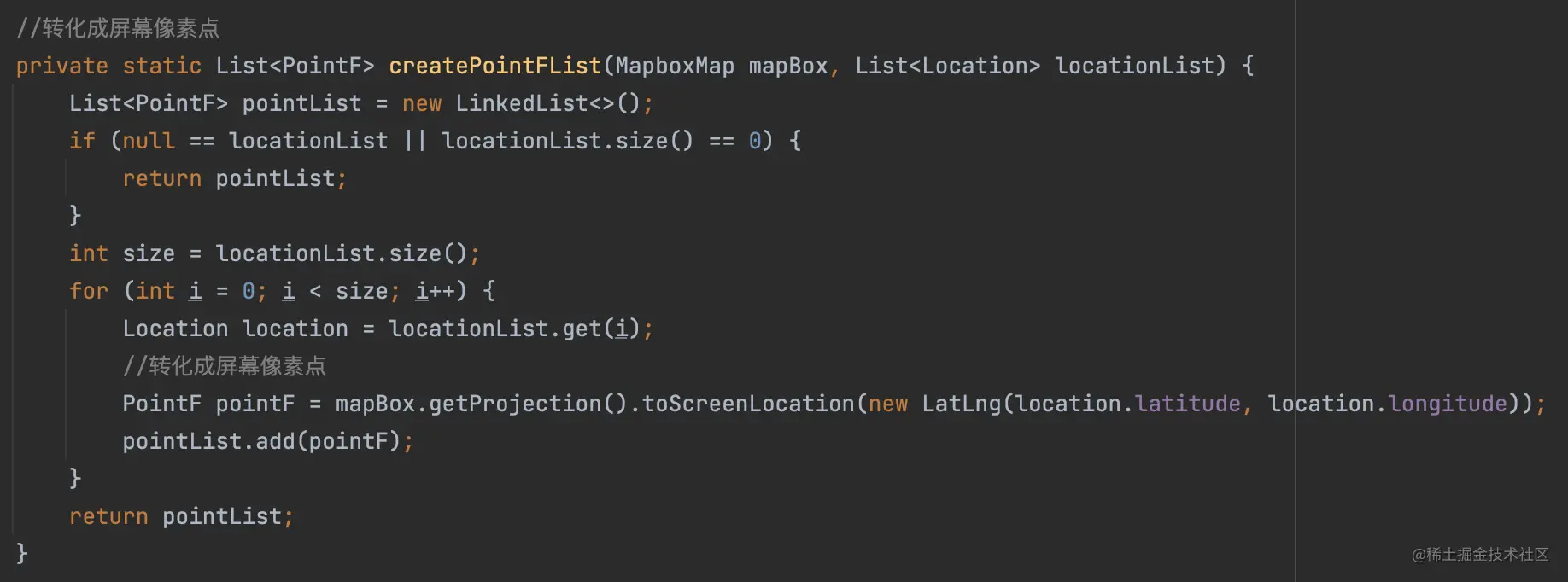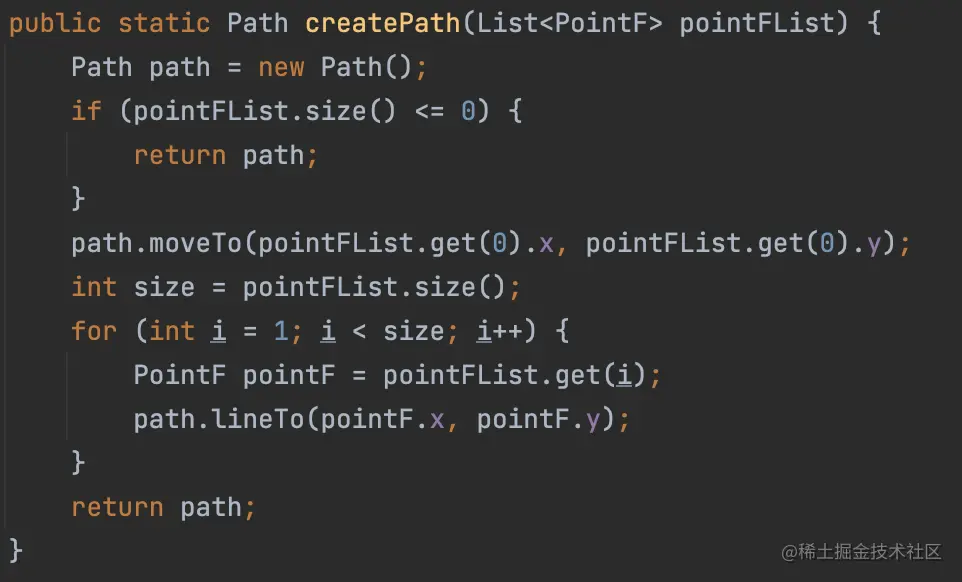• 基于Length做属性动画：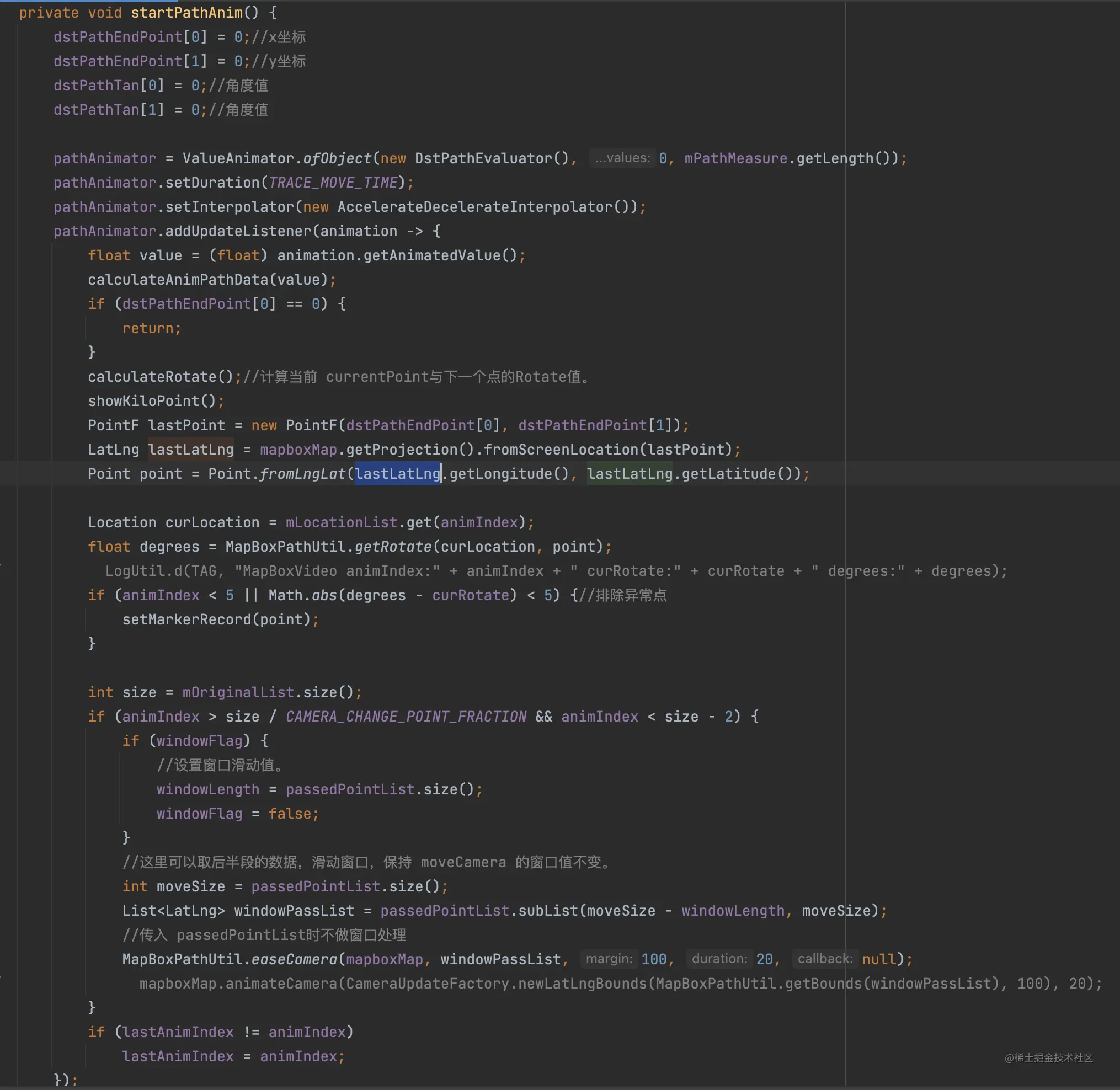``ValueAnimator.ofObject(new DstPathEvaluator(), 0, mPathMeasure.getLength());``

``````dstPathEndPoint = 0;//x坐标
dstPathEndPoint = 0;//y坐标
dstPathTan = 0;//角度值
dstPathTan = 0;//角度值``````

``````PointF lastPoint = new PointF(dstPathEndPoint, dstPathEndPoint);
LatLng lastLatLng = mapboxMap.getProjection().fromScreenLocation(lastPoint);
Point point = Point.fromLngLat(lastLatLng.getLongitude(), lastLatLng.getLatitude());``````

``````Location curLocation = mLocationList.get(animIndex);
float degrees = MapBoxPathUtil.getRotate(curLocation, point);
if (animIndex < 5 || Math.abs(degrees - curRotate) < 5) {//排除异常点
setMarkerRecord(point);
}``````

setMarkerRecord(point) 方法调用加入到 Map 轨迹的绘制Layer中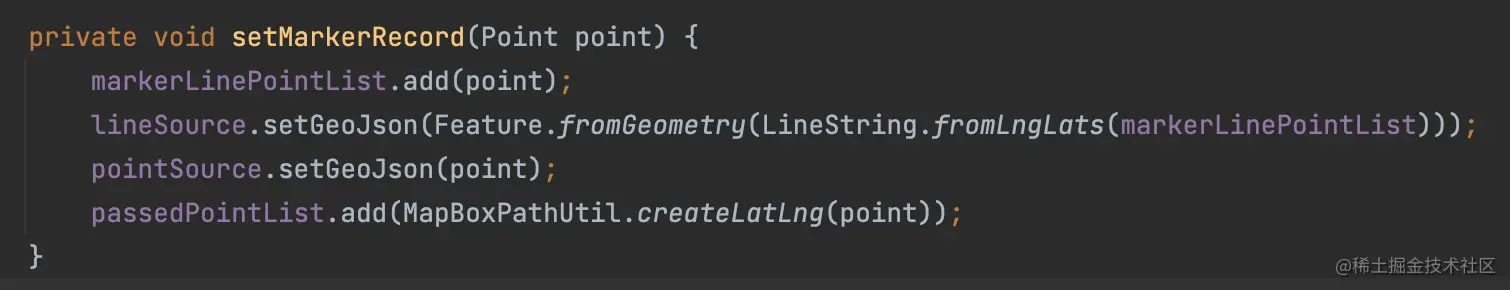``````//这里可以取后半段的数据，滑动窗口，保持 moveCamera 的窗口值不变。
int moveSize = passedPointList.size();
List<LatLng> windowPassList = passedPointList.subList(moveSize - windowLength, moveSize);``````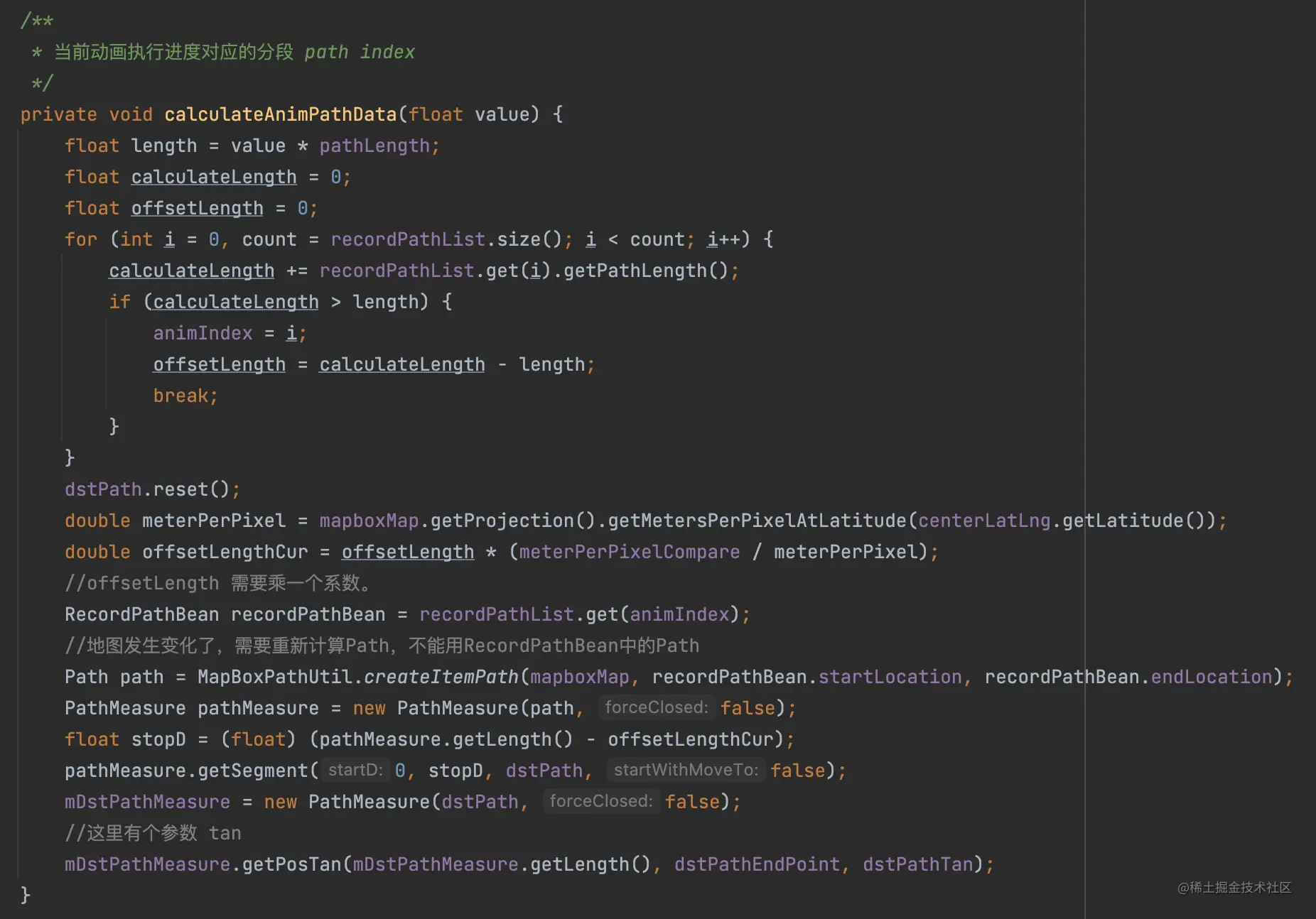``RecordPathBean recordPathBean = recordPathList.get(animIndex);``

``float stopD = (float) (pathMeasure.getLength() - offsetLengthCur);``

``````pathMeasure.getSegment(0, stopD, dstPath, false);
mDstPathMeasure = new PathMeasure(dstPath, false);
//这里有个参数 tan
mDstPathMeasure.getPosTan(mDstPathMeasure.getLength(), dstPathEndPoint, dstPathTan);``````

• 整公里点的绘制